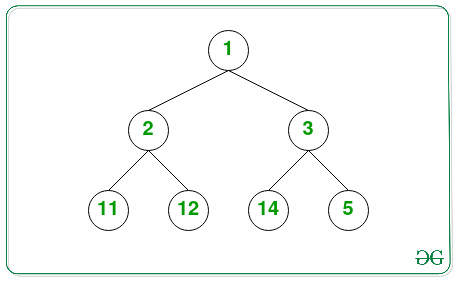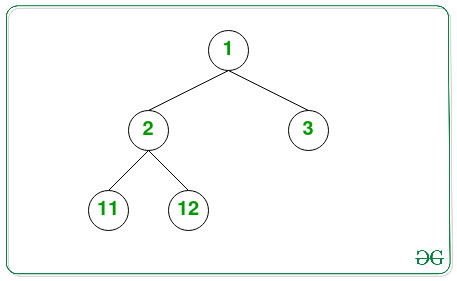# Count number of nodes in a complete Binary Tree

• Difficulty Level : Easy
• Last Updated : 04 Aug, 2022

Given the root of a Complete Binary Tree consisting of N nodes, the task is to find the total number of nodes in the given Binary Tree.

Examples:

Input:Output: 7

Input:Output: 5

Naive Approach: The simple approach to solve the given tree is to perform the DFS Traversal on the given tree and count the number of nodes in it. After traversal, print the total count of nodes obtained.

Time Complexity: O(N) as in traversal all the nodes are visited
Auxiliary Space: O(1)

Efficient Approach: The above approach can also be optimized by the fact that:

A complete binary tree can have at most (2h – 1) nodes in total where h is the height of the tree (This happens when all the levels are completely filled).

By this logic, in the first case, compare the left sub-tree height with the right sub-tree height. If they are equal it is a full tree, then the answer will be 2^height – 1. Otherwise, If they aren’t equal, recursively call for the left sub-tree and the right sub-tree to count the number of nodes. Follow the steps below to solve the problem:

• Define a function left_height(root) and find the left height of the given Tree by traversing in the root’s left direction and store it in a variable, say leftHeight.
• Define a function right_height(root) and find the right height of the given Tree by traversing in the root’s right direction and store it in a variable, say rightHeight.
• Find the left and the right height of the given Tree for the current root value and if it is equal then return the value of (2height – 1) as the resultant count of nodes.
• Otherwise, recursively call for the function for the left and right sub-trees and return the sum of them + 1 as the resultant count of nodes.

Below is the implementation of the above approach.

## C++

 `// C++ program for the above approach ` `#include ` `using` `namespace` `std; ` ` `  `// Structure of a Tree Node ` `class` `node { ` `public``: ` `    ``int` `data; ` `    ``node* left; ` `    ``node* right; ` `}; ` ` `  `node* newNode(``int` `data); ` ` `  `// Function to get the left height of ` `// the binary tree ` `int` `left_height(node* node) ` `{ ` `    ``int` `ht = 0; ` `    ``while` `(node) { ` `        ``ht++; ` `        ``node = node->left; ` `    ``} ` `    ``// Return the left height obtained ` `    ``return` `ht; ` `} ` ` `  `// Function to get the right height ` `// of the binary tree ` `int` `right_height(node* node) ` `{ ` `    ``int` `ht = 0; ` `    ``while` `(node) { ` `        ``ht++; ` `        ``node = node->right; ` `    ``} ` `    ``// Return the right height obtained ` `    ``return` `ht; ` `} ` ` `  `// Function to get the count of nodes ` `// in complete binary tree ` `int` `TotalNodes(node* root) ` `{ ` `    ``// Base Case ` `    ``if` `(root == NULL) ` `        ``return` `0; ` `    ``// Find the left height and the ` `    ``// right heights ` `    ``int` `lh = left_height(root); ` `    ``int` `rh = right_height(root); ` `    ``// If left and right heights are ` `    ``// equal return 2^height(1<left) ` `           ``+ TotalNodes(root->right); ` `} ` ` `  `// Helper function to allocate a new node ` `// with the given data ` `node* newNode(``int` `data) ` `{ ` `    ``node* Node = ``new` `node(); ` `    ``Node->data = data; ` `    ``Node->left = NULL; ` `    ``Node->right = NULL; ` `    ``return` `(Node); ` `} ` ` `  `// Driver Code ` `int` `main() ` `{ ` `    ``node* root = newNode(1); ` `    ``root->left = newNode(2); ` `    ``root->right = newNode(3); ` `    ``root->left->left = newNode(4); ` `    ``root->left->right = newNode(5); ` `    ``root->right->left = newNode(9); ` `    ``root->right->right = newNode(8); ` `    ``root->left->left->left = newNode(6); ` `    ``root->left->left->right = newNode(7); ` `    ``cout << TotalNodes(root); ` `    ``return` `0; ` `} ` ` `  `// This code is contributed by aditya kumar (adityakumar129)`

## C

 `// C program for the above approach ` `#include ` `#include ` ` `  `// Structure of a Tree Node ` `typedef` `struct` `node { ` `    ``int` `data; ` `    ``struct` `node* left; ` `    ``struct` `node* right; ` `}node; ` ` `  `// Helper function to allocate a new node ` `// with the given data ` `node* newNode(``int` `data) ` `{ ` `  ``node * Node = (node *)``malloc``(``sizeof``(node)); ` `    ``Node->data = data; ` `    ``Node->left = NULL; ` `    ``Node->right = NULL; ` `    ``return` `(Node); ` `} ` ` `  `// Function to get the left height of ` `// the binary tree ` `int` `left_height(node* node) ` `{ ` `    ``int` `ht = 0; ` `    ``while` `(node) { ` `        ``ht++; ` `        ``node = node->left; ` `    ``} ` `    ``// Return the left height obtained ` `    ``return` `ht; ` `} ` ` `  `// Function to get the right height ` `// of the binary tree ` `int` `right_height(node* node) ` `{ ` `    ``int` `ht = 0; ` `    ``while` `(node) { ` `        ``ht++; ` `        ``node = node->right; ` `    ``} ` ` `  `    ``// Return the right height obtained ` `    ``return` `ht; ` `} ` ` `  `// Function to get the count of nodes ` `// in complete binary tree ` `int` `TotalNodes(node* root) ` `{ ` `    ``// Base Case ` `    ``if` `(root == NULL) ` `        ``return` `0; ` `    ``// Find the left height and the ` `    ``// right heights ` `    ``int` `lh = left_height(root); ` `    ``int` `rh = right_height(root); ` `    ``// If left and right heights are ` `    ``// equal return 2^height(1<left) + TotalNodes(root->right); ` `} ` ` `  `// Driver Code ` `int` `main() ` `{ ` `    ``node* root = newNode(1); ` `    ``root->left = newNode(2); ` `    ``root->right = newNode(3); ` `    ``root->left->left = newNode(4); ` `    ``root->left->right = newNode(5); ` `    ``root->right->left = newNode(9); ` `    ``root->right->right = newNode(8); ` `    ``root->left->left->left = newNode(6); ` `    ``root->left->left->right = newNode(7); ` `    ``printf``(``"%d"``,TotalNodes(root)); ` `    ``return` `0; ` `} ` ` `  `// This code is contributed by aditya kumar (adityakumar129)`

## Java

 `// Java program for the above approach ` `import` `java.util.*; ` ` `  `class` `GFG{ ` ` `  `// Structure of a Tree Node ` `static` `class` `node { ` ` `  `    ``int` `data; ` `    ``node left; ` `    ``node right; ` `}; ` ` `  ` `  `// Function to get the left height of ` `// the binary tree ` `static` `int` `left_height(node node) ` `{ ` `    ``int` `ht = ``0``; ` `    ``while` `(node!=``null``) { ` `        ``ht++; ` `        ``node = node.left; ` `    ``} ` ` `  `    ``// Return the left height obtained ` `    ``return` `ht; ` `} ` ` `  `// Function to get the right height ` `// of the binary tree ` `static` `int` `right_height(node node) ` `{ ` `    ``int` `ht = ``0``; ` `    ``while` `(node!=``null``) { ` `        ``ht++; ` `        ``node = node.right; ` `    ``} ` ` `  `    ``// Return the right height obtained ` `    ``return` `ht; ` `} ` ` `  `// Function to get the count of nodes ` `// in complete binary tree ` `static` `int` `TotalNodes(node root) ` `{ ` ` `  `    ``// Base Case ` `    ``if` `(root == ``null``) ` `        ``return` `0``; ` ` `  `    ``// Find the left height and the ` `    ``// right heights ` `    ``int` `lh = left_height(root); ` `    ``int` `rh = right_height(root); ` ` `  `    ``// If left and right heights are ` `    ``// equal return 2^height(1<

## Python3

 `# Python program for the above approach ` ` `  `# Structure of a Tree Node ` `class` `node: ` `    ``def` `__init__(``self``, key): ` `        ``self``.left ``=` `None` `        ``self``.right ``=` `None` `        ``self``.val ``=` `key ` ` `  `# Function to get the left height of ` `# the binary tree ` `def` `left_height(node): ` `    ``ht ``=` `0` `    ``while``(node): ` `        ``ht ``+``=` `1` `        ``node ``=` `node.left ` `         `  `     ``# Return the left height obtained ` `    ``return` `ht ` ` `  `# Function to get the right height ` `# of the binary tree ` `def` `right_height(node): ` `    ``ht ``=` `0` `    ``while``(node): ` `        ``ht ``+``=` `1` `        ``node ``=` `node.right ` `         `  `    ``# Return the right height obtained ` `    ``return` `ht ` ` `  `# Function to get the count of nodes ` `# in complete binary tree ` `def` `TotalNodes(root): ` `   `  `  ``# Base case ` `    ``if``(root ``=``=` `None``): ` `        ``return` `0` `       `  `     ``# Find the left height and the ` `    ``# right heights ` `    ``lh ``=` `left_height(root) ` `    ``rh ``=` `right_height(root) ` `     `  `     ``# If left and right heights are ` `    ``# equal return 2^height(1<

## C#

 `// C# program for the above approach ` `using` `System; ` ` `  `public` `class` `GFG{ ` ` `  `// Structure of a Tree Node ` `class` `node { ` ` `  `    ``public` `int` `data; ` `    ``public` `node left; ` `    ``public` `node right; ` `}; ` ` `  ` `  `// Function to get the left height of ` `// the binary tree ` `static` `int` `left_height(node node) ` `{ ` `    ``int` `ht = 0; ` `    ``while` `(node != ``null``) { ` `        ``ht++; ` `        ``node = node.left; ` `    ``} ` ` `  `    ``// Return the left height obtained ` `    ``return` `ht; ` `} ` ` `  `// Function to get the right height ` `// of the binary tree ` `static` `int` `right_height(node node) ` `{ ` `    ``int` `ht = 0; ` `    ``while` `(node != ``null``) { ` `        ``ht++; ` `        ``node = node.right; ` `    ``} ` ` `  `    ``// Return the right height obtained ` `    ``return` `ht; ` `} ` ` `  `// Function to get the count of nodes ` `// in complete binary tree ` `static` `int` `TotalNodes(node root) ` `{ ` ` `  `    ``// Base Case ` `    ``if` `(root == ``null``) ` `        ``return` `0; ` ` `  `    ``// Find the left height and the ` `    ``// right heights ` `    ``int` `lh = left_height(root); ` `    ``int` `rh = right_height(root); ` ` `  `    ``// If left and right heights are ` `    ``// equal return 2^height(1<

## Javascript

 ``

Output

`9`

Time Complexity: O((log N)2)

• Calculating the height of a tree with x nodes takes (log x) time.
• Here, we are traversing through the height of the tree.
• For each node, height calculation takes logarithmic time.
• As the number of nodes is N, we are traversing log(N) nodes and calculating the height for each of them.
• So the total complexity is (log N * log N) = (log N)2.

Auxiliary Space: O(log N)  because of Recursion Stack Space (which takes elements upto the maximum depth of a node in the tree)

My Personal Notes arrow_drop_up
Recommended Articles
Page :